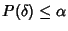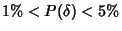## Significance

Let. A valuesuch thatis considered significant'' (i.e., is not simply due to chance) is known as an Alpha Value. The Probability that a variate would assume a value greater than or equal to the observed value strictly by chance,, is known as a P-Value.

Depending on the type of data and conventional practices of a given field of study, a variety of different alpha values may be used. One commonly used terminology takesas not significant,'', as significant'' (sometimes denoted *), andas highly significant'' (sometimes denoted **). Some authors use the term almost significant'' to refer to, although this practice is not recommended.

See also Alpha Value, Confidence Interval, P-Value, Probable Error, Significance Test, Statistical Test